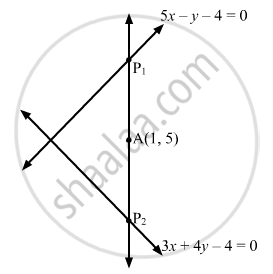Department of Pre-University Education, KarnatakaPUC Karnataka Science Class 11
Advertisement Remove all ads

# A Line is Such that Its Segment Between the Straight Lines 5x − Y − 4 = 0 and 3x + 4y − 4 = 0 is Bisected at the Point (1, 5). Obtain Its Equation. - Mathematics

Answer in Brief

A line is such that its segment between the straight lines 5x − y − 4 = 0 and 3x + 4y − 4 = 0 is bisected at the point (1, 5). Obtain its equation.

Advertisement Remove all ads

#### Solution

Let P1P2 be the intercept between the lines 5x − y − 4 = 0 and 3x + 4y − 4 = 0.
Let P1P2 make an angle $\theta$ with the positive x-axis.

Here,

$\left( x_1 , y_1 \right) = A \left( 1, 5 \right)$

So, the equation of the line passing through A (1, 5) is

$\frac{x - x_1}{cos\theta} = \frac{y - y_1}{sin\theta}$

$\Rightarrow \frac{x - 1}{cos\theta} = \frac{y - 5}{sin\theta}$

$\Rightarrow \frac{y - 5}{x - 1} = \tan\theta$Let $A P_1 = A P_2 = r$

Then, the coordinates of $P_1 \text { and } P_2$ are given by $\frac{x - 1}{cos\theta} = \frac{y - 5}{sin\theta} = r \text { and } \frac{x - 1}{cos\theta} = \frac{y - 5}{sin\theta} = - r$

So, the coordinates of $P_1 \text { and } P_2$ are  $\left( 1 + rcos\theta, 5 + r\sin\theta \right) \text { and } \left( 1 - rcos\theta, 5 - r\sin\theta \right)$ respectively.
Clearly,

$P_1 \text { and } P_2$ lie on 5x − y − 4 = 0 and 3x + 4y − 4 = 0, respectively.

$\therefore 5\left( 1 + rcos\theta \right) - 5 - r\sin\theta - 4 = 0 \text { and } 3\left( 1 - rcos\theta \right) + 4\left( 5 - r\sin\theta \right) - 4 = 0$

$\Rightarrow r = \frac{4}{5cos\theta - sin\theta} \text { and } r = \frac{19}{3cos\theta + 4sin\theta}$

$\Rightarrow \frac{4}{5cos\theta - sin\theta} = \frac{19}{3cos\theta + 4sin\theta}$

$\Rightarrow 95cos\theta - 19sin\theta = 12cos\theta + 16sin\theta$

$\Rightarrow 83cos\theta = 35sin\theta$

$\Rightarrow tan\theta = \frac{83}{35}$

Thus, the equation of the required line is

$\frac{y - 5}{x - 1} = tan\theta$

$\Rightarrow \frac{y - 5}{x - 1} = \frac{83}{35}$

$\Rightarrow 83x - 35y + 92 = 0$

Concept: Straight Lines - Equation of Family of Lines Passing Through the Point of Intersection of Two Lines
Is there an error in this question or solution?
Advertisement Remove all ads

#### APPEARS IN

RD Sharma Class 11 Mathematics Textbook
Chapter 23 The straight lines
Exercise 23.8 | Q 12 | Page 66
Advertisement Remove all ads
Advertisement Remove all ads
Share
Notifications

View all notifications

Forgot password?